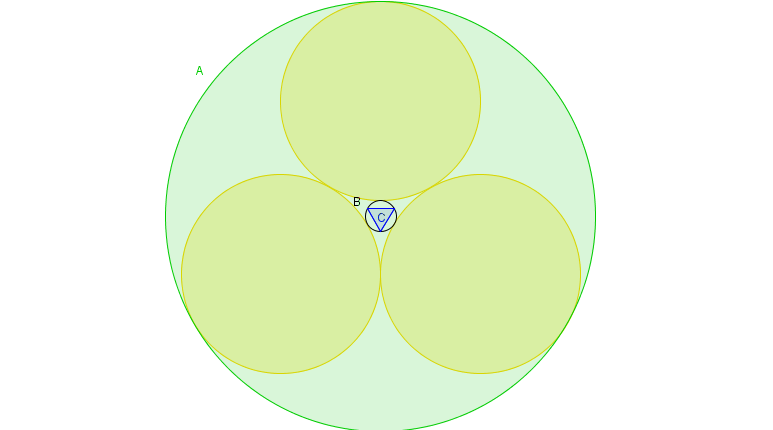# A triangle surrounded by circles

Geometry Level 5In a circle $A$ with radius $\frac{18+\sqrt{432}}{6}$ we draw $3$ circles with equal radii, in such way that each of them are tangent to the other $2$ circles and to circle $A$. Inside the circle $A$ we draw a circle $B$ in such way that is tangent to each of the $3$ new circles. An equilateral triangle $C$ is inscribed in $B$.

If the area of $C$ can be written in the form:

$\dfrac{a\sqrt{b}-c}{d}$

Where $a,b,c$ and $d$ are integers such that $b|a$ and $d|c$.

Find:

$a+b+c+d$

×